﻿ 先进战斗机全动V尾抖振动强度设计与验证Download PDF文章快速检索 高级检索

1. 中国航空工业成都飞机设计研究所, 成都 610091;
2. 西北工业大学 航空学院, 西安 710072;
3. 中国空气动力研究与发展中心, 绵阳 621000;
4. 中国飞机强度研究所, 西安 710065

Strength desigh and test of advanced fighter all-moving twin V-tail buffet
JIN Wei1, YANG Zhichun2, MENG Dehong3, CHEN Yan1, HUANG Hu1, WANG Yongjun1, HE Shi4, CHEN Yuanfang1
1. AVIC Chengdu Aircraft Design and Research Institute, Chengdu 610091, China;
2. School of Aeronautics, Northwestern Polytechnical University, Xi'an 710072, China;
3. China Aerodynamics Research and Development Center, Mianyang 621000, China;
4. China Aircraft Strength Research Institute, Xi'an 710065, China
Abstract: High Angle of Attack (AoA) maneuverability is the symbolic characteristic of the fourth generation fighter. Our advanced fighter utilizes twin V-tails configuration, and this sort of aerodynamic design is proved to be well controllable at high AoA maneuvering flight. During high AoA maneuvering flight, the strong flow disturbance induced by the burst vortex shedding from fore-body impinge inevitably on the twin V-tails, and will induce severe buffet. The V-tails buffet will cause not only remarkable reduction of flight quality, but also structural fatigue damage, leading to high maintenance cost. This paper describes in detail the key techniques achieved during the engineering manufacturing and design of an aircraft with all-moving twin V-tails, which include: the design technique of rigid/ flexible hybrid model and testing methods of all-moving V-tail buffet wind tunnel test, as well as the principle of similarity transformation method which transforms test data from buffet wind tunnel test to fighter flight condition; the CFD/CSD coupling calculation method based on the RANS/LES hybrid algorithm; active control technique on V-tail buffet response alleviation based on macro fiber composite piezoelectric actuators and Positive Acceleration Feedback (PAF); the V-tail buffet fatigue spectrum generation method and test program. This research has established a set of systematical theoretical analysis techniques, design criteria, and test methods for aircraft V-tail structure buffet dynamic strength design and test of advanced fighters and UAVs.
Keywords: buffet    buffet spectrum    dynamic fatigue    wind tunnel test    shedding vortex    macro fiber composite

1 全动V尾抖振风洞试验“刚性/弹性”组合模型的风洞试验方法

V尾布局先进战斗机在做大迎角机动飞行时，尤其是在跨声速区进行盘旋和收敛转弯机动时，前机身分离的脱体涡破裂后打到V尾上会导致V尾发生严重的抖振。破裂的高强度脱体涡包含有各种尺度的非线性混合涡流，频率成份复杂，采用数值仿真方法获得抖振载荷的时间周期较长，在型号的初步设计阶段，完成V尾抖振动强度分析所需要的翼面加速度响应和脉动压力动态载荷主要依靠风洞试验获取。对于高机动性先进战斗机其飞行包线远远超出抖振起始边界，抖振风洞试验中需要弄清在这些飞行状态下V尾结构的抖振动响应与动态载荷。在V尾布局抖振模型风洞试验中，通常将一侧V尾模型设计成刚性模型，在其翼面两侧布置成对的贴片式脉动压力传感器测量时域脉动压力差，再转换到飞机尺度作为V尾结构动强度计算的动态载荷输入。而另一侧V尾模型则设计成动力相似(或结构相似)的弹性模型。弹性模型可以在风洞试验中直观地反映翼面抖振响应随迎角、速压的变化趋势，直接验证抖振响应减缓控制措施的效果，测量的动响应和V尾根部弯矩、扭矩等动态载荷还能够直接按照相似比例尺转换得到飞机尺度结构的动响应量值以及动态内力载荷。因此，在型号研制中，分别进行了全机低速与跨声速的V尾“刚性/弹性”组合模型的抖振风洞试验，给出了把抖振风洞试验动态测试结果向飞机尺度进行相似转换的原理与方法，主要研究工作体现在以下几个方面。

1.1 V尾弹性/刚性抖振模型风洞试验结果向飞机尺度相似转换的原理与方法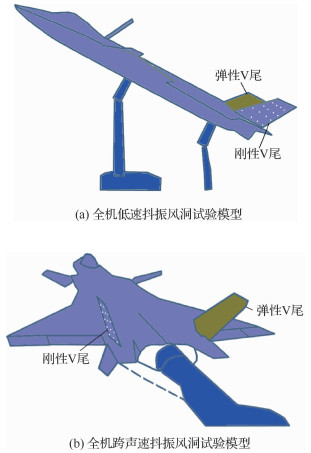图 1 全机状态V尾抖振风洞试验(刚/弹)组合模型 Fig. 1 Complete aircraft V-tail buffet (rigid/ elastic) hybrid model for wind tunnel test

 ${{\eta _{\rm{m}}} = \frac{{{\omega _{\rm{m}}}{L_{\rm{m}}}}}{{{V_{\rm{m}}}}} = \frac{{{\omega _{\rm{a}}}{L_{\rm{a}}}}}{{{V_{\rm{a}}}}} = {\eta _{\rm{a}}}}$ （1）
 ${{K_\omega } = \frac{1}{{{K_L}}} \cdot \frac{{{V_{\rm{m}}}}}{{{V_{\rm{a}}}}} = \frac{1}{{{K_L}}}{{({K_q}/{K_\rho })}^{\frac{1}{2}}}}$ （2）

 ${{K_\omega } = \frac{{{{({K_q}/{K_\rho })}^{\frac{1}{2}}}}}{{{K_L}}}}$ （3）

 ${{\varphi _{\rm{a}}} = {{({L_{\rm{a}}}/{L_{\rm{m}}})}^4}{{({\rho _{\rm{a}}}/{\rho _{\rm{m}}})}^2}{{({V_{\rm{a}}}/{V_{\rm{m}}})}^3}{\varphi _{\rm{m}}}}$

 ${{K_{{\rm{CP}}}} = (K_{\rm{m}}^2K_\omega ^3)/K_L^2 = {K_L}{{({K_\rho }K_q^3)}^{1/2}}}$

 ${{K_{{\rm{ap}}}} = K_L^2K_\omega ^3 = {{({K_q}/{K_\rho })}^{3/2}}/{K_L}}$

 ${{K_{{\rm{MP}}}} = K_L^4K_{\rm{m}}^2K_\omega ^3 = K_L^7K_\rho ^{1/2}K_q^{3/2}}$

1.2 低速、高速抖振风洞试验V尾翼面脉动压力相关性分析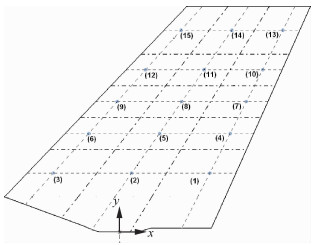图 2 V尾翼面脉动压力传感器布置 Fig. 2 Locations of V-tail fluctuating pressure transducers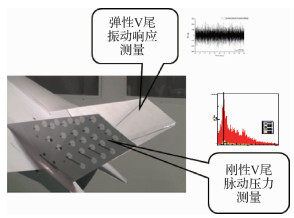图 3 抖振风洞试验刚/弹性V尾模型的数据测量 Fig. 3 Data measurement of rigid/elastic V-tail model in buffet wind tunnel test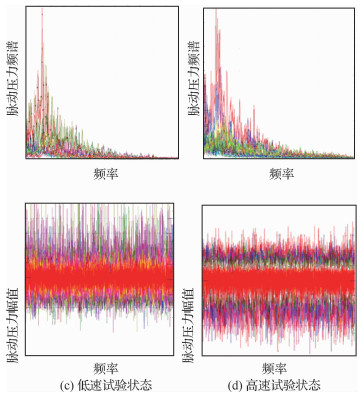图 4 低速、高速风洞试验结果比较 Fig. 4 Comparison between low and high speed wind tunnel test results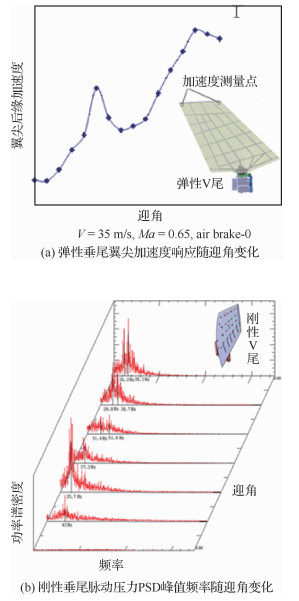图 5 全机状态V尾(刚/弹)组合模型抖振风洞试验结果 Fig. 5 Buffet wind tunnel test results of complete aircraft V-tail (rigid/ elastic) hybrid model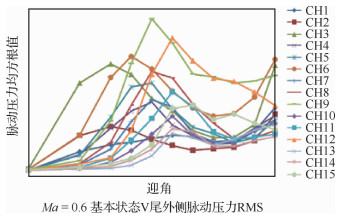图 6 V尾翼面内、外侧脉动压力分布随迎角变化 Fig. 6 Pressure distribution vs angle of attack at inside and outside of V-tail
2 基于RANS/LES混合算法进行V尾结构抖振响应的CFD/CSD耦合计算方法 2.1 基于DES混合算法的脱体涡流场计算方法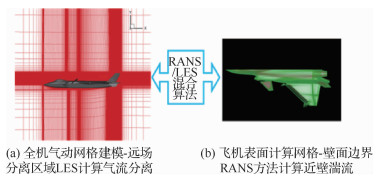图 7 RANS/LES混合算法气动模型 Fig. 7 RANS/LES hybrid algorithm aerodynamic model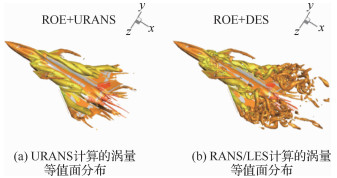图 8 URANS与RANS/LES计算结果对比 Fig. 8 Compare of URANS and RANS/LES results (Ma=0.3、迎角30°) (Ma=0.3, angle of attack 30°)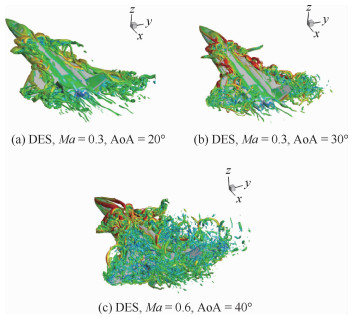图 9 不同迎角状态下脱体涡流场空间分布 Fig. 9 Detached vortex flow field spatial distribution with different angle of attack
2.2 V尾结构抖振响应CFD/CSD耦合计算方法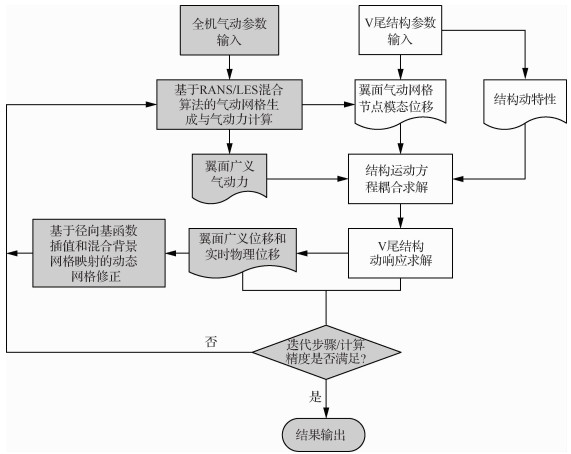图 10 V尾抖振响应CFD/CSD高效高精度计算方法与流程 Fig. 10 CFD/CSD efficient and high precision calculation method and flowchart for V-tail buffet response

 马赫数 迎角/(°) 翼尖后缘加速度响应均方根值误差/% 0.6 20 -4.7 0.8 20 -5.2 0.3 30 3.4 0.6 30 -6.3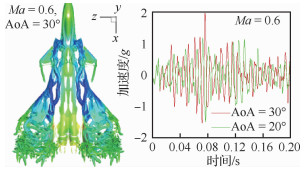图 11 V尾翼尖广义坐标下的加速度响应 Fig. 11 Acceleration response of V-tail in generalized coordinates
3 基于正加速度反馈的V尾抖振响应压电控制技术

PAF控制方法具有以下优点：①不需要精细的控制系统模型，只需根据系统的固有频率和相位关系设计控制补偿器；②控制系统通过引入二阶补偿器构成新的闭环系统，可以根据劳斯-赫尔维茨稳定性判据或李雅普诺夫稳定性判据获得系统稳定边界，指导控制器参数的选择；③在具体实施时，可以通过适当地选取增益确定系统的稳定裕度，使得当抖振载荷作用于V尾结构造成系统参数变动的情况下，依然能够获得良好的控制效果，控制的自适应能力较强；④控制系统通过补偿器辅助系统与被控对象构成新的闭环系统，可在结构的主要固有频率处大幅提高系统的阻尼比，直接降低结构的抖振响应，同时辅助系统的引入并不会对原系统的稳定性造成影响。

3.1 正加速度反馈控制方法的建立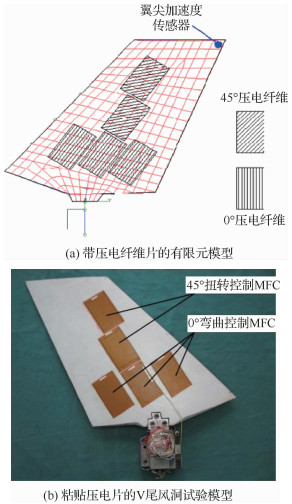图 12 V尾翼面压电纤维与传感器布置 Fig. 12 Arrangement of V-tail piezoelectric fiber and sensor

 ${\ddot x + 2\zeta {\omega _{\rm{n}}}\dot x + \omega _{\rm{n}}^2x = u}$ （4）

 ${\left\{ {\begin{array}{*{20}{l}} {\ddot x + 2{\zeta _{\rm{f}}}{\omega _{\rm{f}}}{\kern 1pt} {\kern 1pt} \dot z + \omega _{\rm{f}}^2{\kern 1pt} z = g{\kern 1pt} \ddot x}\\ {u = g{\kern 1pt} \ddot z} \end{array}} \right.}$ （5）

 $\begin{array}{l} \left[ {\begin{array}{*{20}{c}} 1&{ - g}\\ { - g}&1 \end{array}} \right]\left[ {\begin{array}{*{20}{c}} {\ddot x}\\ {\ddot z} \end{array}} \right] + \left[ {\begin{array}{*{20}{c}} {2\zeta {\omega _{\rm{n}}}}&0\\ 0&{2{\zeta _{\rm{f}}}{\omega _{\rm{f}}}} \end{array}} \right]\left[ {\begin{array}{*{20}{c}} {\dot x}\\ {\dot z} \end{array}} \right] + \\ {\kern 1pt} {\kern 1pt} {\kern 1pt} {\kern 1pt} {\kern 1pt} {\kern 1pt} {\kern 1pt} {\kern 1pt} {\kern 1pt} {\kern 1pt} {\kern 1pt} {\kern 1pt} {\kern 1pt} {\kern 1pt} {\kern 1pt} {\kern 1pt} {\kern 1pt} {\kern 1pt} {\kern 1pt} \left[ {\begin{array}{*{20}{c}} {\omega _n^2}&0\\ 0&{\omega _{\rm{f}}^2} \end{array}} \right]\left[ {\begin{array}{*{20}{l}} x\\ z \end{array}} \right] = \left[ {\begin{array}{*{20}{l}} 0\\ 0 \end{array}} \right] \end{array}$ （6）

 ${0 < g < 1}$ （7）

 ${\frac{{X(s)}}{{Z(s)}} = \frac{{g{s^2}}}{{{s^2} + 2{\zeta _{\rm{f}}}{\omega _{\rm{f}}}s + \omega _{\rm{f}}^2}}}$ （8）

3.2 基于PAF控制方法的控制系统设计与仿真

PAF控制方法除了可以增大闭环系统的阻尼比外，还可通过对V尾结构响应贡献最大的一弯模态和一扭模态设计独立的控制器与补偿器，使闭环控制器的传递函数在设计点处(V尾一阶弯曲频率和一阶扭转频率)的相位与被控系统传递函数在该点处的相位相反，这样来达到降低结构抖振响应的目的。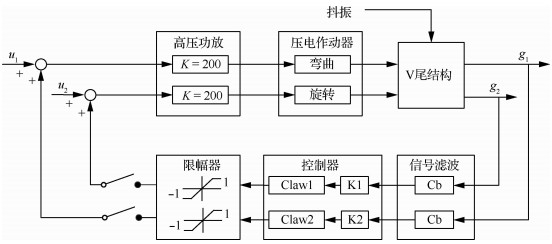图 13 V尾模型抖振响应PAF控制系统设计框图 Fig. 13 Block diagram of PAF control system for V-tail model buffet response

2个反馈通道信号的传递函数在设计点处的相位与被控系统(辨识系统)传递函数相位对比见图 14，在此点处控制系统实际获得的相位差均接近反相要求的180°。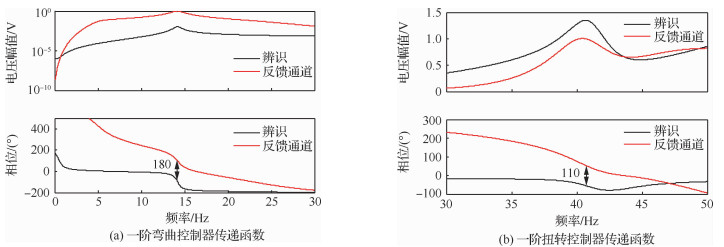图 14 控制系统传递函数相位与被控系统(辨识系统)传递函数相位对比 Fig. 14 Comparison of transfer function phases between control system and controlled system (recognized system)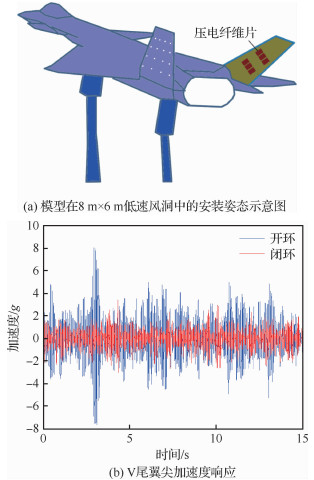图 15 先进战斗机V尾抖振响应控制低速风洞试验 Fig. 15 Low speed wind tunnel test for advanced fighter V-tail buffet response control
4 V尾抖振动态疲劳载荷谱编制方法与试验实施方案

4.1 V尾抖振频率分布与AoA-sf参数表计算

V尾抖振刚性模型风洞试验用于测量垂尾翼面的脉动压力分布。在翼面各个分区的内、外侧翼面对位布置压力传感器，测量脉动压力差并进行谱分析。然后按照以下公式转换到飞机尺度

 ${\frac{{{\varphi _{\rm{m}}}({\omega _{\rm{m}}})}}{{{{(1/2{\rho _{\rm{m}}}V_{\rm{m}}^2)}^2}}}\left( {\frac{{{V_{\rm{m}}}}}{{{L_{\rm{m}}}}}} \right) = \frac{{{\varphi _{\rm{a}}}({\omega _{\rm{a}}})}}{{{{(1/2{\rho _{\rm{a}}}V_{\rm{a}}^2)}^2}}}\left( {\frac{{{V_{\rm{a}}}}}{{{L_{\rm{a}}}}}} \right)}$ （9）

 AoA/(°) f/Hz q=10~30 kPa q=20~50 kPa q=50~70 kPa q=70~90 kPa 18 16.0 21.0 42.0 65.0 30 15.5 18.2 40.3 63.0
4.2 V尾抖振AoA-q-t参数表计算

 AoA/(°) t/s q=10~30 kPa q=20~50 kPa q=50~70 kPa q=70~90 kPa 18 2 500.0 2 000.0 4560.0 2 651.0 30 2 800.0 2 400.0 4 800.0 2 726.0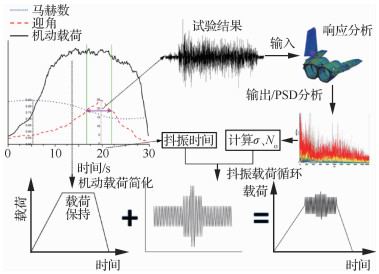图 16 机动载荷叠加抖振载荷方法示意图 Fig. 16 Sketch map of superposition of maneuver-load and buffet-load
4.3 抖振载荷循环的简化原理

 ${f({x_a}) = \frac{{{x_a}}}{{{\sigma ^2}}}{{\rm{e}}^{ - \frac{1}{2}{{\left( {\frac{{x_a^2}}{\sigma }} \right)}^2}}}{\kern 1pt} {\kern 1pt} {\kern 1pt} {\kern 1pt} {\kern 1pt} {\kern 1pt} {\kern 1pt} {\kern 1pt} {\kern 1pt} {\kern 1pt} {\kern 1pt} {\kern 1pt} {\kern 1pt} {x_a} > 0}$ （10）

Rayleigh分布如图 17所示，σ取响应时间历程的均方根值(RMS)作为估计量。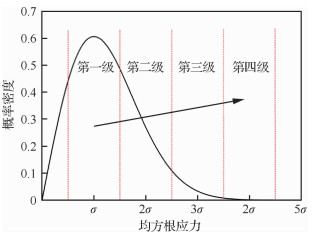图 17 Rayleigh分布 Fig. 17 Rayleigh distribution

 ${{D_{{\rm{ buff }}}} = T\frac{{{N_0}}}{C}\int_0^\infty {{f_a}} ({x_a}){{({x_a})}^n}{\rm{d}}{x_a}}$ （11）

 ${{D_{{\rm{ buff }}}} = T\frac{{{N_0}}}{C}{\sigma ^n}\int_0^\infty {{y^{n + 1}}} {{\rm{e}}^{ - \frac{{{y^2}}}{2}}}{\rm{d}}y}$ （12）

 $\left\{ \begin{array}{l} T\frac{{{N_0}}}{C}{\sigma ^n}\int_{1.5}^{2.5} {{y^{n + 1}}} {{\rm{e}}^{ - \frac{{{y^2}}}{2}}}{\rm{d}}y = \frac{{{N_{p2\sigma }}}}{C} \cdot {(2\sigma )^n}\\ T\frac{{{N_0}}}{C}{\sigma ^n}\int_{2.5}^{3.5} {{y^{n + 1}}} {{\rm{e}}^{ - \frac{{{y^2}}}{2}}}{\rm{d}}y = \frac{{{N_{p3\sigma }}}}{C} \cdot {(3\sigma )^n}\\ T\frac{{{N_0}}}{C}{\sigma ^n}\left( {\int_{0.5}^{1.5} {{y^{n + 1}}} {{\rm{e}}^{ - \frac{{{y^2}}}{2}}}{\rm{d}}y + } \right.\\ {\kern 1pt} {\kern 1pt} {\kern 1pt} {\kern 1pt} {\kern 1pt} {\kern 1pt} {\kern 1pt} {\kern 1pt} {\kern 1pt} {\kern 1pt} {\kern 1pt} {\kern 1pt} {\kern 1pt} {\kern 1pt} {\kern 1pt} {\kern 1pt} {\kern 1pt} {\kern 1pt} {\kern 1pt} {\kern 1pt} {\kern 1pt} {\kern 1pt} {\kern 1pt} {\kern 1pt} {\kern 1pt} {\kern 1pt} {\kern 1pt} \left. {\int_{3.5}^{4.5} {{y^{n + 1}}} {{\rm{e}}^{ - \frac{{{y^2}}}{2}}}{\rm{d}}y} \right) = \frac{{{N_{p4\sigma }}}}{C} \cdot {(4\sigma )^n} \end{array} \right.$ （13）

 $\left\{ {\begin{array}{*{20}{l}} {{N_{p{\kern 1pt} 2\sigma }} = 0.25T{N_0}}\\ {{N_{p{\kern 1pt} 3\sigma }} = 0.033{\kern 1pt} {\kern 1pt} {\kern 1pt} 5T{N_0}}\\ {{N_{p{\kern 1pt} 4\sigma }} = 0.005T{N_0}} \end{array}} \right.$ （14）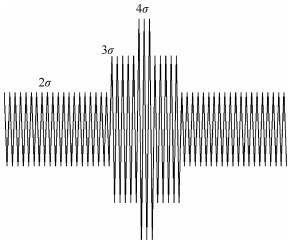图 18 简化后的抖振谱 Fig. 18 Simplified buffet load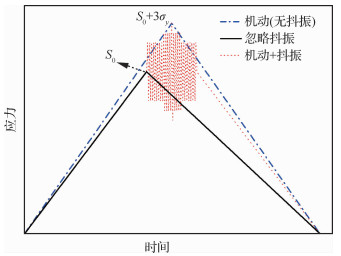图 19 机动载荷叠加抖振载荷方法示意图 Fig. 19 Sketch of superposition methods of maneuver load and buffet load

1) 整个过程不发生抖振，只有机动载荷循环(黑色实线)。

2) 抖振发生，但抖振载荷循环的损伤可忽略，考虑叠加形成的最大载荷循环(蓝色虚线)。

3) 考虑最大载荷叠加的载荷循环，同时抖振不可忽略，等效为等幅循环(虚线)。

4.4 考虑叠加机动载荷和抖振载荷谱编制的应用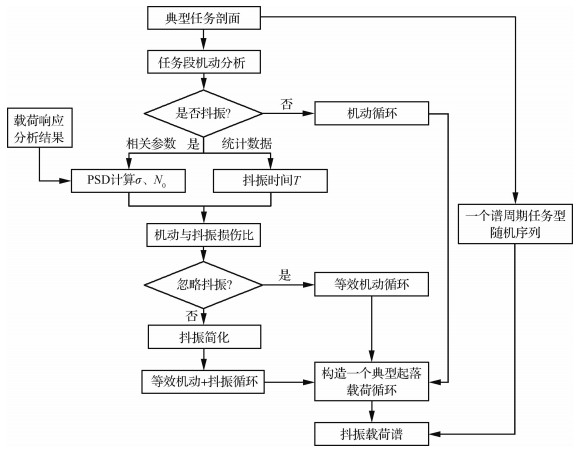图 20 抖振和机动载荷叠加的编谱流程 Fig. 20 Flowchart of spectrum produce of maneuver-load and buffet-load superposition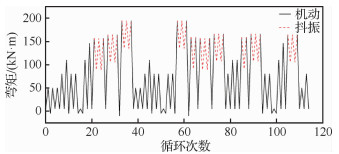图 21 一个完整起落的典型疲劳载荷循环谱 Fig. 21 Typical load cyclic spectrum of one take-off and landing
4.5 V尾抖振疲劳试验方案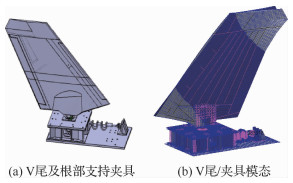图 22 V尾动力学相似支持夹具与垂尾模态 Fig. 22 V-tail dynamic similar fixture and the calculated V-tail mode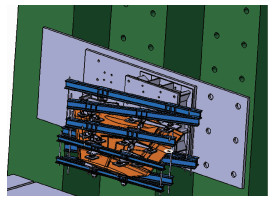图 23 常规机动疲劳试验加载系统 Fig. 23 Loading system for conventional maneuver fatigue test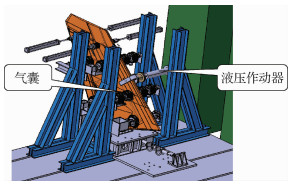图 24 叠加机动静载的抖振疲劳试验加载系统 Fig. 24 Loading system for buffet fatigue test superposition with maneuver static load
5 结论

  LEE B H K, BROWN D. Wind tunnel studies of F/A-18 tail buffet[J]. Journal of Aircraft, 1992, 29(1): 146-152. Click to display the text  李劲杰, 杨青, 肖春生, 等. 边条翼布局流场及其双垂尾抖振特性研究[J]. 航空学报, 2006, 27(3): 395-398. LI J J, YANG Q, XIAO C S, et al. Investigation of flow and twin-vertical tail buffet characteristics of leading-edge extension configuration[J]. Acta Aeronautica et Astronautica Sinica, 2006, 27(3): 395-398. (in Chinese) Cited By in Cnki (10) | Click to display the text  HEALEY M D. F/A-18 E/F vertical tail buffet design, analysis and test[C]//44th AIAA/ASME/ASCE/AHS/ASC Structures, Structural Dynamics, and Materials Conference. Reston: AIAA, 2003.  CHRIS M. Incorporation of buffet into the F-35 full scale durability tests spectra[R].F-35-Structural Integrity Program, 2006.  CHRISTONPHER L B. Buffet fatigue sequence generation from F-22 flight test data using frequency domain methods[C]//48th AIAA/ASME/ASCE/AHS/ASC Structures, Structural Dynamics, and Materials Conference.Reston: AIAA, 2007.  PATEL S R. F/A-22 vertical tail buffet strength certification[C]//46th AIAA/ASME/ASCE/AHS/ASC Structures, Structural Dynamics and Materials Conference. Reston: AIAA, 2005.  YEHIA M R. Numerical investigation of tail buffet on F-18 aircraft[C]//10th Applied Aerodynamics Conference, 1992.  KEN G. Computational analysis of FA-18 tail buffet[C]//20th Atmospheric Flight Mechanics Conference, 1995.  BRAFORD E G. Computational prediction of nose-down control for F/A-18E at high alpha[J]. Journal of Aircraft, 2008, 45(5): 1661-1668. Click to display the text  王巍.压电结构振动主动控制及在尾翼抖振响应控制中的应用[D].西安: 西北工业大学, 2009: 30-35. WANG W. Application of piezoelectric structural vibration active control and its application to the control of vertical tail buffeting response[D]. Xi'an: Northwestern Polytechnical University, 2009: 30-35(in Chinese).  MORES R W. Active control of F/A-18 vertical tail buffeting using piezoelectric actuators[C]//44th AIAA/ASME/ASCE/AHS/ASC Structures, Structural Dynamics, and Materials Conference.Reston: AIAA, 2003.  ASHLEY H. Further study of active control for fin buffet alleviation with application to F-22: WL-TR-97-3073[R]. 1997.  WILLIAM D A. F/A-22 high angle of attack buffet-test adequacy analysis[C]//22nd Applied Aerodynamics Conference and Exhibit Providence, 2004.  HEWWITT R L. Spectrum editing for a full-scale fatigue test of a fighter aircraft wing with buffet loading[C]//ASTM Special Technical Publication, Fatigue Testing and Analysis Under Variable Amplitude Loading Conditions, 2003.  CONSER D P, KEYS G L. F/A-18 production ASPJ vertical tail dynamic fatigue test FT98 test spectra development: MDC 91B0424[R]. St.LouiS: McDonnell Douglas Corporation, 1992.  HEALEY M D. F/A-18E/F vertical tail dynamic fatigue test ESD03 test spectra development: MDC 93B0168[R]. St. Loui S: McDonnell Douglas Corporation, 1995.  MOLENT L A D. Buffet loads and structural redundancy considerations inertial tail fatigue monitoring programs[C]//International Aerospace Congress, 1999.  SHETA E F. Control of twin-tail buffet responses using normal leading-edge blowing: AIAA-2000-4227[R].Reston: AIAA, 2000.  SHETA E F, HUTTSELL L J. Numerical analysis of F/A-18 vertical tail buffeting[C]//19th AIAA Applied Aerodynamics Conference Anaheim.Reston: AIAA, 2001.  FERMAN M A, PAGEL S R. A unified approach to buffet response of fighter aircraft empennage: AGARD Report CP-483[R]. Sorrento: North Atlantic Treaty Organization, 1990.  SHAH G H, GRAFTON S B, GUYNN M D, et al. Effect of vortex flow characteristics on tail buffet and high angle of attack aerodynamics of a twin tail fighter configuration[C]//High Angle of Attack Technology Conference.Washington, D.C.: NASA, 1990.  ANDERSON W D, PATEL S R, BLACK C L. Low speed wind tunnel buffet testing on the F/A-22[C]//45th AIAA/ASME/ASCE/AHS/ASC Structures, Structural Dynamics and Materials Conference. Reston: AIAA, 2004.
http://dx.doi.org/10.7527/S1000-6893.2019.23473

0

#### 文章信息

JIN Wei, YANG Zhichun, MENG Dehong, CHEN Yan, HUANG Hu, WANG Yongjun, HE Shi, CHEN Yuanfang

Strength desigh and test of advanced fighter all-moving twin V-tail buffet

Acta Aeronautica et Astronautica Sinica, 2020, 41(6): 523473.
http://dx.doi.org/10.7527/S1000-6893.2019.23473